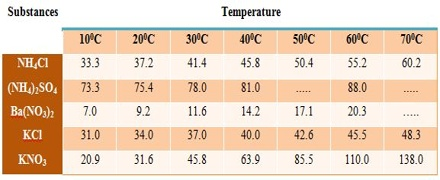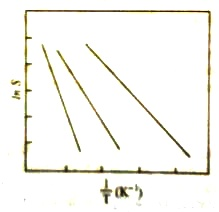# Effect of Temperature on Solubility in Liquid Solution

Effect of Temperature on Solubility

When solids dissolve in a liquid heat is generally absorbed, i.e., dissolution of a solid in a liquid is an endothermic process. The solubility of such solids increases with the increase of temperature in accord with Le Chatelier principle. When dissolution is accompanied by evolution of heat the solubility is found to decrease with rise of temperature, again in agreement with the Le Chatelier principle.Figure: Solubility as a function of temperature

The solubility’s of a few solids in water at different temperatures are given in Figure and Table.

Table: Solubility of salts at different temperatures (g solute / 100 g H2O)By treating solubility as an equilibrium constant, one can deduce the relation between solubility and temperature, e.g.,

(d Ln S / dT) = (∆H / RT2)

Ln S = – (∆H / RT) + constant … … … (1)

where S is the solubility at temperature T and ∆H is the enthalpy of solution. Equation (1) may be integrated within limits to the following form:

Ln (S2/S1) = – (∆H / RT) (1/T2 – 1/T1) … … … (2)

According to equation (2) a plot of Ln S against 1/T should give a straight line (Figure 2) with a slope of (∆H / T). If the solubility values at different temperatures are known, the heat of solution can be calculated from the slope of the straight lines as in Figure 2.Equation (2) may be used to calculate the value of ∆H from solubility values at any two temperatures from equation (2) it can be seen that the temperature effect on solubility depends on the heat of solution. Breaks in the solubility curves are named in the case at salts which exist in different hydrated forms as for example, sodium sulphate (Figure 1). The first part is the solubility curve for sodium sulphate decahydrate (Na2SO4.10H2O) while the higher temperature pan is that for the unhydrated salt. This difference in solubility behavior may be traced to difference in the heats of solution of the two forms. Transition temperatures of hydrated salts may thus be obtained from solubility data.